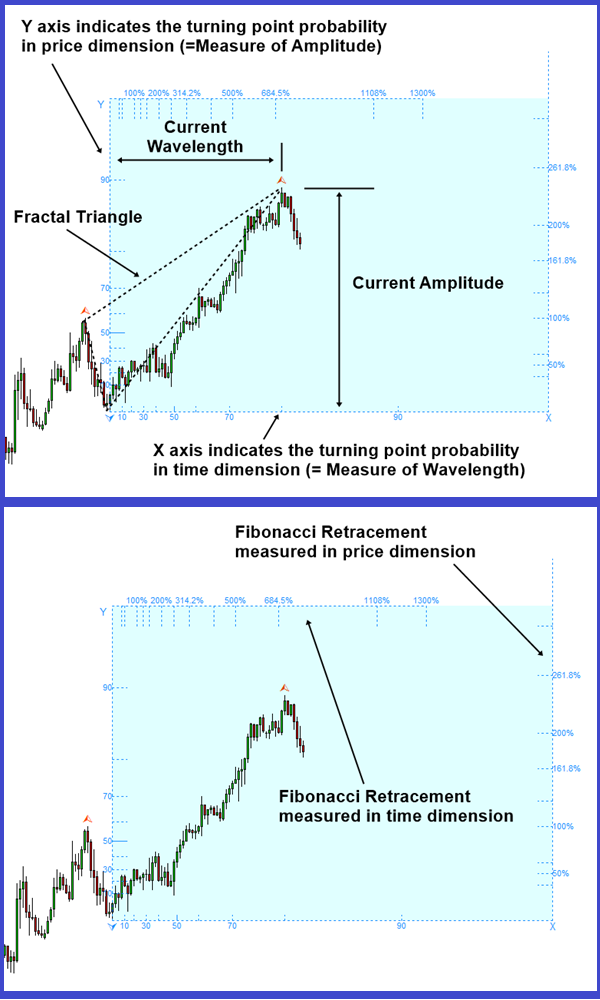# Science of Fibonacci Analysis in Forex and Stock Market

Fibonacci Analysis in Forex and Stock market is an important technique for your trading although it is hard to find out any scientific reference to its usage. However, for sure, you will often find that the price reaction around the Fibonacci ratio is the key to successful trading as well as the profit taking. At the same time, understanding the Fibonacci analysis open up your knowledge gate for Harmonic Pattern, Elliott Wave and X3 Chart Pattern as well as other price pattern.

A Fibonacci analysis is a popular tool among technical traders. It is based on the Fibonacci sequence numbers identified by Leonardo Fibonacci, the Italian mathematician, in the 13th century. The Fibonacci sequence numbers are: 0, 1, 1, 2, 3, 5, 8, 13, 21, 34, 55, 89,144, 233, 377, 610, 987, 1597, 2584, 4181, 6765, ………………… As the Fibonacci number become large, the constant relationship is established between neighbouring numbers. The relationship converges to the Golden ratio, 0.618 (or 1.618).

Although the Fibonacci Analysis are the key to successful trading, no book or article will really pin down the science behind the Fibonacci Analysis. The book: Science Of Support, Resistance, Fibonacci Analysis, Harmonic Pattern, Elliott Wave and X3 Chart Pattern (In Forex and Stock Market Trading) is exactly the book dedicated for this topic. This book will tell you the untold story of the Fibonacci Analysis, which will become the valuable wisdom for Forex and Stock market trading.

Understanding the scientific principle always help you to bring it further than other traders. In this book, we shows the Fibonacci Retracement in price dimension and Fibonacci Retracement in time dimension. Using the Fibonacci-Probability Graph, you will find the ways to combine the turning point probability with the Fibonacci Retracement in price dimension and Fibonacci Retracement in time dimension.

The book can be found in all the major book distributors in the world. Please choose the best book distributor you like from the link below including amazon.com, Google Play Book, scribd.com, Apple Book and so on.

https://www.amazon.com/dp/B0993WZGZD

Fibonacci-Probability graph is exclusively available from Fractal Pattern Scanner in MetaTrader 4 and MetaTrader 5. It could be used to identify the turning point and breakout opportunity. Below is the landing page for Fractal Pattern Scanner in MetaTrader version.

https://www.mql5.com/en/market/product/49170

https://www.mql5.com/en/market/product/49169